By Michael Lewin

Boolean operators are the bedrock of computer logic. Michael Lewin investigates a common one and shows there’s more to it than meets the eye.

You probably already know what XOR is, but let’s take a moment to formalise it. XOR is one of the sixteen possible binary operations on Boolean operands. That means that it takes 2 inputs (it’s binary) and produces one output (it’s an operation), and the inputs and outputs may only take the values of TRUE or FALSE (it’s Boolean) – see Figure 1. We can (and will) interchangeably consider these values as being 1 or 0 respectively, and that is why XOR is typically represented by the symbol ⊕: it is equivalent to the addition operation on the integers modulo 2 (i.e. we wrap around so that 1 + 1 = 0) 1 [ SurreyUni ]. I will use this symbol throughout, except in code examples where I will use the C operator ``` ^ ``` to represent XOR.

XOR Truth Table
Input A Input B Output
0 0 0
0 1 1
1 0 1
1 1 0
Figure 1

Certain Boolean operations are analogous to set operations (see Figure 2): AND is analogous to intersection, OR is analogous to union, and XOR is analogous to set difference. This is not just a nice coincidence; mathematically it is known as an isomorphism 2 and it provides us with a very neat way to visualise and reason about such operations.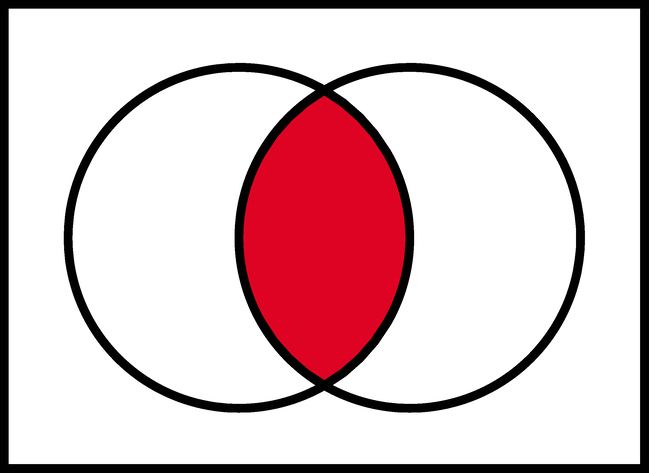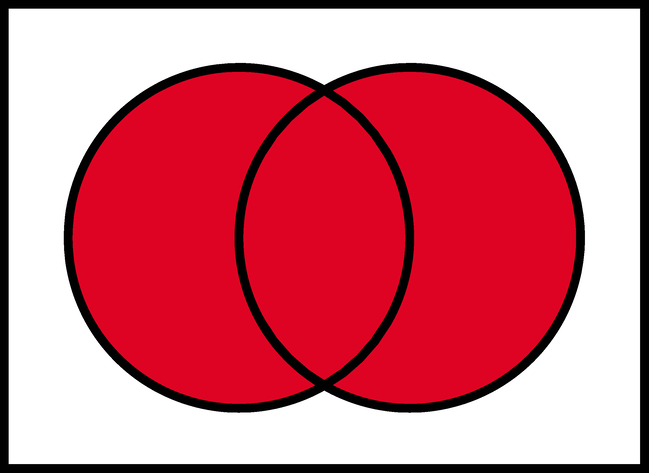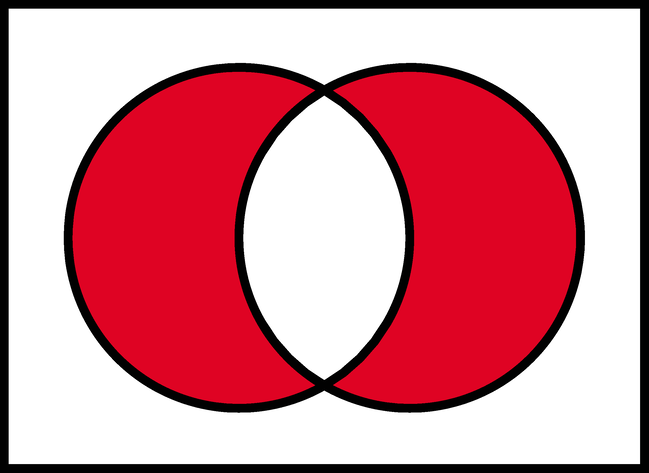Figure 2

## Important properties of XOR

There are 4 very important properties of XOR that we will be making use of. These are formal mathematical terms but actually the concepts are very simple.

1. Commutative : A ⊕ B = B ⊕ A

This is clear from the definition of XOR: it doesn’t matter which way round you order the two inputs.

2. Associative : A ⊕ ( B ⊕ C ) = ( A ⊕ B ) ⊕ C

This means that XOR operations can be chained together and the order doesn’t matter. If you aren’t convinced of the truth of this statement, try drawing the truth tables.

3. Identity element : A ⊕ 0 = A

This means that any value XOR’d with zero is left unchanged.

4. Self-inverse : A ⊕ A = 0

This means that any value XOR’d with itself gives zero.

These properties hold not only when XOR is applied to a single bit, but also when it is applied bitwise to a vector of bits (e.g. a byte). For the rest of this article I will refer to such vectors as bytes, because it is a concept that all programmers are comfortable with, but don’t let that make you think that the properties only apply to a vector of size 8.

## Interpretations

We can interpret the action of XOR in a number of different ways, and this helps to shed light on its properties. The most obvious way to interpret it is as its name suggests, ‘exclusive OR’: A ⊕ B is true if and only if precisely one of A and B is true. Another way to think of it is as identifying difference in a pair of bytes: A ⊕ B = ‘the bits where they differ’. This interpretation makes it obvious that A ⊕ A = 0 (byte A does not differ from itself in any bit) and A ⊕ 0 = A (byte A differs from 0 precisely in the bit positions that equal 1) and is also useful when thinking about toggling and encryption later on.

The last, and most powerful, interpretation of XOR is in terms of parity , i.e. whether something is odd or even. For any n bits, A1 ⊕ A2 ⊕ … ⊕ A n = 1 if and only if the number of 1s is odd. This can be proved quite easily by induction and use of associativity. It is the crucial observation that leads to many of the properties that follow, including error detection, data protection and adding.

## Toggling

Armed with these ideas, we are ready to explore some applications of XOR. Consider the following simple code snippet:

```  for (int n=x; true; n ^= (x ^ y))
printf("%d ", n);```

This will toggle between two values ``` x ``` and ``` y ``` , alternately printing one and then the other. How does it work? Essentially the combined value ``` x ^ y ``` ‘remembers’ both states, and one state is the key to getting at the other. To prove that this is the case we will use all of the properties covered earlier:

 B ⊕ ( A ⊕ B ) (commutative ) = B ⊕ ( B ⊕ A ) (associative) = (B ⊕ B) ⊕ A (self-inverse) = 0 ⊕ A (identity element) = A

Toggling in this way is very similar to the concept of a flip-flop in electronics: a ‘circuit that has two stable states and can be used to store state information’ [ Wikipedia-1 ].

## Save yourself a register

Toggling is all very well, but it’s probably not that useful in practice. Here’s a function that is more useful. If you haven’t encountered it before, see if you can guess what it does.

```  void s(int& a, int& b)
{
a = a ^ b;
b = a ^ b;
a = a ^ b;
}```

Did you work it out? It’s certainly not obvious, and the below equivalent function is even more esoteric:

```  void s(int& a, int& b)
{
a ^= b ^= a ^= b;
}```

It’s an old trick that inspires equal measures of admiration and vilification. In fact there is a whole repository of interview questions whose name is inspired by this wily puzzle: http://xorswap.com/. That’s right, it’s a function to swap two variables in place without having to use a temporary variable. Analysing the first version: the first line creates the XOR’d value. The second line comprises an expression that evaluates to ``` a ``` and stores it in ``` b ``` , just as the toggling example did. The third line comprises an expression that evaluates to ``` b ``` and stores it in ``` a ``` . And we’re done! Except there’s a bug: what happens if we call ``` s(myVal, myVal) ``` ? This is an example of aliasing , where two arguments to a function share the same location in memory, so altering one will affect the other. The outcome is that ``` myVal == 0 ``` which is certainly not the semantics we expect from a swap function!

Perhaps there is some retribution for this much maligned idea, however. This is more than just a devious trick when we consider it in the context of assembly language. In fact XOR’ing a register with itself is the fastest way for the compiler to zero the register.

A node in a singly linked list contains a value and a pointer to the next node. A node in a doubly linked list contains the same, plus a pointer to the previous node. But in fact it’s possible to do away with that extra storage requirement. Instead of storing either pointer directly, suppose we store the XOR’d value of the previous and next pointers [ Wikipedia-2 ] – see Figure 3.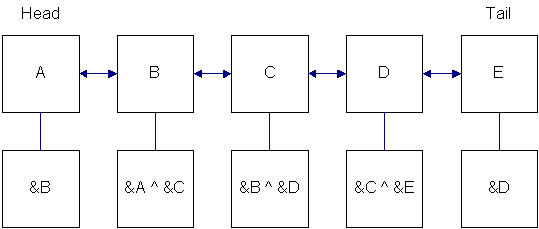Figure 3

Note that the nodes at either end store the address of their neighbours. This is consistent because conceptually we have XOR’ed that address with 0. Then the code to traverse the list looks like Listing 1, which was adapted from Stackoverflow [ Stackoverflow ].

 ```// traverse the list given either the head or // the tail void traverse( Node *endPoint ) { Node* prev = endPoint; Node* cur = endPoint; while ( cur ) // loop until we reach a null pointer { printf( "value = %d\n", cur->value); if ( cur == prev ) // only true on first iteration cur = cur->prevXorNext; // move to next node in the list else { Node* temp = cur; cur = (Node*)((uintptr_t)prev ^ (uintptr_t)cur->prevXorNext); // move to next node in the list prev = temp; } } } ``` Listing 1

This uses the same idea as before, that one state is the key to getting at the other. If we know the address of any consecutive pair of nodes, we can derive the address of their neighbours. In particular, by starting from one end we can traverse the list in its entirety. A nice feature of this function is that this same code can be used to traverse either forwards or backwards. One important caveat is that it cannot be used in conjunction with garbage collection, since by obfuscating the nodes’ addresses in this way the nodes would get marked as unreachable and so could be garbage collected prematurely.

## Pseudorandom number generator

XOR can also be used to generate pseudorandom numbers in hardware. A pseudorandom number generator (whether in hardware or software e.g. ``` std::rand() ``` ) is not truly random; rather it generates a deterministic sequence of numbers that appears random in the sense that there is no obvious pattern to it. This can be achieved very fast in hardware using a linear feedback shift register . To generate the next number in the sequence, XOR the highest 2 bits together and put the result into the lowest bit, shifting all the other bits up by one. This is a simple algorithm but more complex ones can be constructed using more XOR gates as a function of more than 2 of the lowest bits [ Yikes ]. By choosing the architecture carefully, one can construct it so that it passes through all possible states before returning to the start of the cycle again (Figure 4).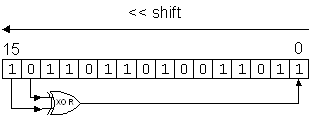Figure 4

## Encryption

The essence of encryption is to apply some key to an input message in order to output a new message. The encryption is only useful if it is very hard to reverse the process. We can achieve this by applying our key over the message using XOR (see Listing 2).

 ```string EncryptDecrypt(string inputMsg,string key) { string outputMsg(inputMsg); short unsigned int keyLength = key.length(); short unsigned int strLength = inputMsg.length(); for(int v=0, k=0;v

The choice of key here is crucial to the strength of the encryption. If it is short, then the code could easily be cracked using the centuries-old technique of frequency analysis. As an extreme example, if the key is just 1 byte then all we have is a substitution cipher that consistently maps each letter of the alphabet to another one. However, if the key is longer than the message, and generated using a ‘ truly random ’ hardware random number generator, then the code is unbreakable [ Wikipedia-3 ]. In practice, this ‘ truly random ’ key could be of fixed length, say 128 bits, and used to define a linear feedback shift register that creates a pseudorandom sequence of arbitrary length known as a keystream . This is known as a stream cipher , and in a real-worl situation this would also be combined with a secure hash function such as md5 or SHA-1 .

Another type of cipher is the block cipher which operates on the message in blocks of fixed size with an unvarying transformation. An example of XOR in this type of encryption is the International Data Encryption Algorithm (IDEA) [ Wikipedia-4 ].

The best-known encryption method is the RSA algorithm . Even when the above algorithm is made unbreakable, it has one crucial disadvantage: it is not a public key system like RSA. Using RSA, I can publish the key others need to send me encrypted messages, but keep secret my private key used to decrypt them. On the other hand, in XOR encryption the same key is used to encrypt and decrypt (again we see an example of toggling). Before you can send me encrypted messages I must find a way to secretly tell you the key to use. If an adversary intercepts that attempt, my code is compromised because they will be able to decrypt all the messages you send me.

## Error detection

Now we will see the first application of XOR with respect to parity . There are many ways to defend against data corruption when sending digital information. One of the simplest is to use XOR to combine all the bits together into a single parity bit which gets appended to the end of the message. By comparing the received parity bit with the calculated one, we can reliably determine when a single bit has been corrupted (or indeed any odd number of bits). But if 2 bits have been corrupted (or indeed any even number of bits) this check will not help us.

Checksums and cyclic redundancy checks (CRC) extend the concept to longer check values and reducing the likelihood of collisions and are widely used. It’s important to note that such checks are error-detecting but not error-correcting : we can tell that an error has occurred, but we don’t know where it occurred and so can’t recover the original message. Examples of error-correcting codes that also rely on XOR are BCH and Reed-Solomon [ Wikipedia-5 ][ IEEEXplore ].

## RAID data protection

The next application of XOR’s parity property is RAID (Redundant Arrays of Inexpensive Disks) [ Mainz ] [ DataClinic ]. It was invented in the 1980s as a way to recover from hard drive corruption. If we have n hard drives, we can create an additional one which contains the XOR value of all the others:

A* = A 1 ⊕ A 2 ⊕ … ⊕ A n

This introduces redundancy : if a failure occurs on one drive, say A1, we can restore it from the others since:

 A 2 ⊕ … ⊕ A n ⊕ A* = A 2 ⊕ … ⊕ A n ⊕ (A 1 ⊕ A 2 ⊕ … ⊕ A n ) (definition of A*) = A 1 ⊕ (A 2 ⊕ A 2 ) ⊕… ⊕ (A n ⊕ A n ) (commutative and associative: rearrange terms) = A 1 ⊕ 0 ⊕… ⊕ 0 (self-inverse) = A 1 (identity element)

This is the same reasoning used to explain toggling earlier, but applied to n inputs rather than just 2.

In the (highly unlikely) event that 2 drives fail simultaneously, the above would not be applicable so there would be no way to recover the data.

## Building blocks of XOR

Let’s take a moment to consider the fundamentals of digital computing, and we will see that XOR holds a special place amongst the binary logical operations.

Computers are built from logic gates, which are in turn built from transistors. A transistor is simply a switch that can be turned on or off using an electrical signal (as opposed to a mechanical switch that requires a human being to operate it). So for example, the AND gate can be built from two transistors in series, since both switches must be closed to allow current to flow, whereas the OR gate can be built from two transistors in parallel, since closing either switch will allow the current to flow.

Most binary logical operations can be constructed from two or fewer transistors; of all 16 possible operations, the only exception is XOR (and its complement, XNOR, which shares its properties). Until recently, the simplest known way to construct XOR required six transistors [ Hindawi ]: the simplest way to see this is in the diagram below, which comprises three gates, each of which requires two transistors. In 2000, Bui et al came up with a design using only four transistors [ Bui00 ] – see Figure 5.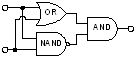Figure 5

## Linear separability

Another way in which XOR stands apart from other such operations is to do with linear separability . This is a concept from Artificial Intelligence relating to classification tasks. Suppose we have a set of data that fall into two categories. Our task is to define a single boundary line (or, extending the notion to higher dimensions, a hyperplane) that neatly partitions the data into its two categories. This is very useful because it gives us the predictive power required to correctly classify new unseen examples. For example, we might want to identify whether or not someone will default on their mortgage payments using only two clues: their annual income and the size of their property. Figure 6 is a hypothetical example of how this might look.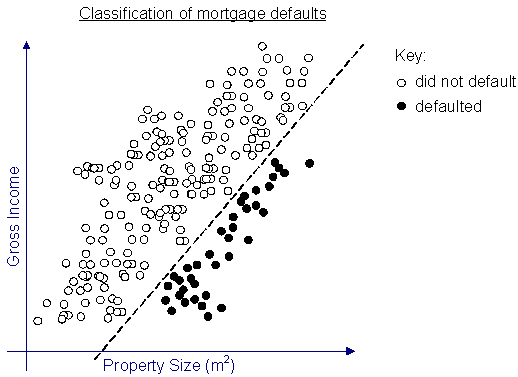Figure 6

A new mortgage application might be evaluated using this model to determine whether the applicant is likely to default.

Not all problems are neatly separable in this way. That means we either need more than one boundary line, or we need to apply some kind of non-linear transformation into a new space in which it is linearly separable: this is how machine learning techniques such as neural networks and support vector machines work. The transformation process might be computationally expensive or completely unachievable. For example, the most commonly used and rigorously understood type of neural network is the multi-layer perceptron . With a single layer it is only capable of classifying linearly separable problems. By adding a second layer it can transform the problem space into a new space in which the data is linearly separable, but there’s no guarantee on how long it may take to converge to a solution.

So where does XOR come into all this? Let’s picture our binary Boolean operations as classification tasks, i.e. we want to classify our four possible inputs into the class that outputs TRUE and the class that outputs FALSE. Of all the 16 possible binary Boolean operations, XOR is the only one (with its complement, XNOR) that is not linearly separable with a single boundary line: two lines are required, as the diagram in Figure 7 demonstrates.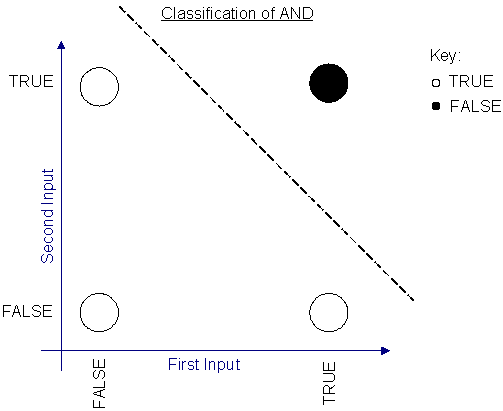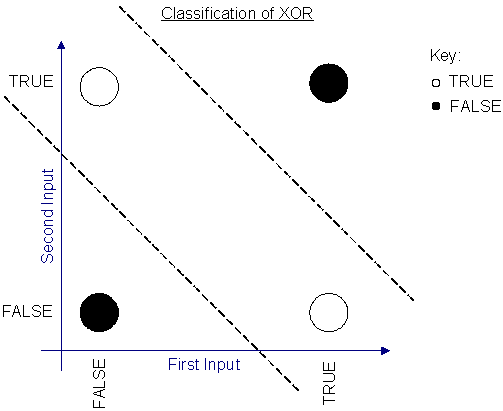Figure 7

XOR also plays a key role inside your processor’s arithmetic logic unit (ALU). We’ve already seen that it is analogous to addition modulo 2, and in fact that is exactly how your processor calculates addition too. Suppose first of all that you just want to add 2 bits together, so the output is a number between 0 and 2. We’ll need two bits to represent such a number. The lower bit can be calculated by XOR’ing the inputs. The upper bit (referred to as the ‘carry bit’) can be calculated with an AND gate because it only equals 1 when both inputs equal 1. So with just these two logic gates, we have a module that can add a pair of bits, giving a 2-bit output. This structure is called a half adder and is depicted in Figure 8.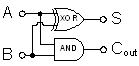Figure 8

Now of course we want to do a lot more than just add two bits: just like you learnt in primary school, we need to carry the ‘carry bit’ along because it will play a part in the calculation of the higher order bits. For that we need to augment what we have into a full adder . We’ve added a third input that enables us to pass in a carry bit from some other adder. We begin with a half adder to add our two input bits. Then we need another half adder to add the result to the input carry bit. Finally we use an OR gate to combine the carry bits output by these two half adders into our overall output carry bit. (If you’re not convinced of this last step, try drawing the truth table.) This structure is represented in Figure 9.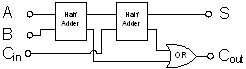Figure 9

Now we are able to chain as many of these adders together as we wish in order to add numbers of any size. The diagram below shows an 8-bit adder array, with the carry bits being passed along from one position to the next. Everything in electronics is modular, so if you want to add 32-bit numbers you could buy four of these components and connect them together (see Figure 10).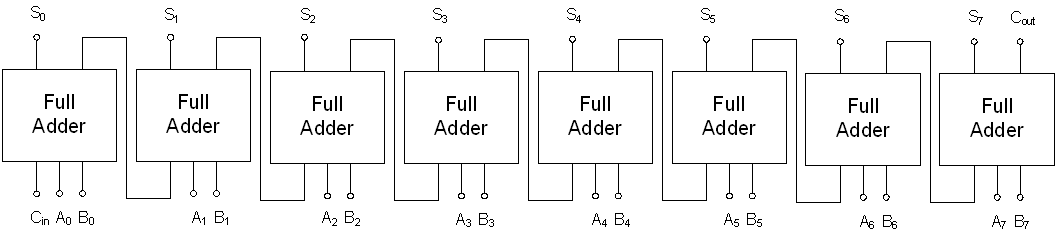Figure 10

If you are interested in learning more about the conceptual building blocks of a modern computer, Charles Petzold’s book Code comes highly recommended.

## More detail on the Group Theory

For those comfortable with the mathematics, here is a bit more detail of how XOR fits into group theory.

An algebraic structure is simply a mathematical object (S, ~) comprising a set S and a binary operation ~ defined on the set.

A group is an algebraic structure such that the following 4 properties hold:

1. ~ is closed over X, i.e. the outcome of performing ~ is always an element of X
2. ~ is associative
3. An identity element e exists that, when combined with any other element of X, leaves it unchanged
4. Every element in X has some inverse that, when combined with it, gives the identity element

We are interested in the operation XOR as applied to the set of Boolean vectors S = {T, F} N , i.e. the set of vectors of length N whose entries can only take the values T and F. (I mean vector in the mathematical sense, i.e. it has fixed length. Do not confuse this with the C++ data structure ``` std::vector ``` , which has variable length.)We have already seen that XOR is associative, that the vector (F, … F) is the identity element and that every element has itself as an inverse. It’s easy to see that it is also closed over the set. Hence (S, XOR) is a group. In fact it is an Abelian group because we showed above that XOR is also commutative.

Two groups are said to be isomorphic if there is a one-to-one mapping between the elements of the sets that preserves the operation. I won’t write that out formally (it’s easy enough to look up) or prove the isomorphisms below (let’s call that an exercise for the reader). Instead I will just define them and state that they are isomorphisms.

The group ({T, F} N , XOR) is isomorphic to the group ({0, 1} N , +) of addition modulo 2 over the set of vectors whose elements are integers mod 2. The isomorphism simply maps T to 1 and F to 0.

The group ({T, F} N , XOR) is also isomorphic to the group (P(S), Δ) of symmetric difference Δ over the power set of N elements 3 : the isomorphism maps T to ‘included in the set’ and F to ‘excluded from the set’ for each of the N entries of the Boolean vector.

Let’s take things one step further by considering a new algebraic structure called a ring . A ring (S,+, ×) comprises a set S and a pair of binary operations + and × such that S is an Abelian group under + and a semigroup 4 under ×. Also × is distributive over +. The symbols + and × are chosen deliberately because these properties mean that the two operations behave like addition and multiplication.

We’ve already seen that XOR is an Abelian group over the set of Boolean vectors, so it can perform the role of the + operation in a ring. It turns out that AND fulfils the role of the * operation. Furthermore we can extend the isomorphisms above by mapping AND to multiplication modulo 2 and set intersection respectively. Thus we have defined three isomorphic rings in the spaces of Boolean algebra, modulo arithmetic and set theory.

## References

[Bui00] H. T. Bui, A. K. Al-Sheraidah, and Y. Wang, ‘New 4-transistor XOR and XNOR designs’, in Proceedings of the 2nd IEEE Asia Pacific Conference

1. In this way, complex logical expressions can be reasoned about and simplified using modulo arithmetic. This is much easier than the commonly taught method of using Karnaugh maps, although OR operations do not map neatly in this way.
2. Formally, the actions of XOR and AND on {0,1} N form a ring that is isomorphic to the actions of set difference and union on sets. For more details see the appendix.
3. The power set means the set of all possible subsets, i.e. this is the set of all sets containing up to N elements.
4. A semigroup is a group without the requirement that every element has an inverse.